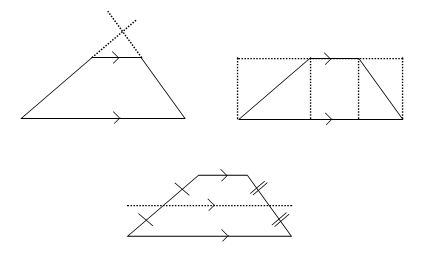Home > Geometric reasoning > Misunderstandings > Revealing the invisible

# Revealing the invisible

Drawing an extra (or auxiliary) line in a diagram can reveal 'invisible' relationships. This strategy is particularly helpful when deriving the properties of quadrilaterals, the angle sum of a triangle, the angle sums of polygons and area formulae.

For example, there are several different auxiliary lines that could be added to a trapezium diagram when deriving the formula for its area. Some are illustrated below.Although some are helpful, others are not. It is valuable for students to explore each situation and determine its merits.

Experimenting with different solution strategies provides students with models for adding auxiliary lines. This helps them see relationships in diagrams beyond the obvious so that they can introduce auxiliary lines without prompting.

A good example of adding extra construction lines can be found when proving the angle sum of a triangle.

You can download the Two Proofs of the Angle Sum of a Triangle PDF. There are further ideas in the Helping to visualise part of the resource.

## An angle in the bend

The 'angle in the bend' activity requires the addition of an auxiliary line in order to solve it.

## Approaching a geometric problem

Knowing where to begin is a common difficulty. Providing a checklist of initial strategies can help students engage with problems.